# TensorFlow 模型建立与训练¶

• 模型的构建： tf.keras.Modeltf.keras.layers

• 模型的损失函数： tf.keras.losses

• 模型的优化器： tf.keras.optimizer

• 模型的评估： tf.keras.metrics

## 模型（Model）与层（Layer）¶

Keras 有两个重要的概念： 模型（Model）层（Layer） 。层将各种计算流程和变量进行了封装（例如基本的全连接层，CNN 的卷积层、池化层等），而模型则将各种层进行组织和连接，并封装成一个整体，描述了如何将输入数据通过各种层以及运算而得到输出。在需要模型调用的时候，使用 y_pred = model(X) 的形式即可。Keras 在 tf.keras.layers 下内置了深度学习中大量常用的的预定义层，同时也允许我们自定义层。

Keras 模型以类的形式呈现，我们可以通过继承 tf.keras.Model 这个 Python 类来定义自己的模型。在继承类中，我们需要重写 __init__() （构造函数，初始化）和 call(input) （模型调用）两个方法，同时也可以根据需要增加自定义的方法。

class MyModel(tf.keras.Model):
def __init__(self):
super().__init__()     # Python 2 下使用 super(MyModel, self).__init__()
# 此处添加初始化代码（包含 call 方法中会用到的层），例如
# layer1 = tf.keras.layers.BuiltInLayer(...)
# layer2 = MyCustomLayer(...)

def call(self, input):
# 此处添加模型调用的代码（处理输入并返回输出），例如
# x = layer1(input)
# output = layer2(x)
return output

# 还可以添加自定义的方法


import tensorflow as tf

X = tf.constant([[1.0, 2.0, 3.0], [4.0, 5.0, 6.0]])
y = tf.constant([[10.0], [20.0]])

class Linear(tf.keras.Model):
def __init__(self):
super().__init__()
self.dense = tf.keras.layers.Dense(
units=1,
activation=None,
kernel_initializer=tf.zeros_initializer(),
bias_initializer=tf.zeros_initializer()
)

def call(self, input):
output = self.dense(input)
return output

# 以下代码结构与前节类似
model = Linear()
optimizer = tf.keras.optimizers.SGD(learning_rate=0.01)
for i in range(100):
y_pred = model(X)      # 调用模型 y_pred = model(X) 而不是显式写出 y_pred = a * X + b
loss = tf.reduce_mean(tf.square(y_pred - y))
print(model.variables)


Keras 的全连接层：线性变换 + 激活函数

• units ：输出张量的维度；

• activation ：激活函数，对应于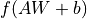中的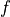，默认为无激活函数（ a(x) = x ）。常用的激活函数包括 tf.nn.relutf.nn.tanhtf.nn.sigmoid

• use_bias ：是否加入偏置向量 bias ，即中的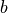。默认为 True

• kernel_initializerbias_initializer ：权重矩阵 kernel 和偏置向量 bias 两个变量的初始化器。默认为 tf.glorot_uniform_initializer 1 。设置为 tf.zeros_initializer 表示将两个变量均初始化为全 0；

1

Keras 中的很多层都默认使用 tf.glorot_uniform_initializer 初始化变量，关于该初始化器可参考 https://www.tensorflow.org/api_docs/python/tf/glorot_uniform_initializer

2

## 基础示例：多层感知机（MLP）¶

• 使用 tf.keras.datasets 获得数据集并预处理

• 使用 tf.keras.Modeltf.keras.layers 构建模型

• 构建模型训练流程，使用 tf.keras.losses 计算损失函数，并使用 tf.keras.optimizer 优化模型

• 构建模型评估流程，使用 tf.keras.metrics 计算评估指标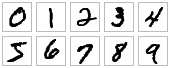MNIST 手写体数字图片示例

### 数据获取及预处理： tf.keras.datasets¶

class MNISTLoader():
def __init__(self):
mnist = tf.keras.datasets.mnist
(self.train_data, self.train_label), (self.test_data, self.test_label) = mnist.load_data()
# MNIST中的图像默认为uint8（0-255的数字）。以下代码将其归一化到0-1之间的浮点数，并在最后增加一维作为颜色通道
self.train_data = np.expand_dims(self.train_data.astype(np.float32) / 255.0, axis=-1)      # [60000, 28, 28, 1]
self.test_data = np.expand_dims(self.test_data.astype(np.float32) / 255.0, axis=-1)        # [10000, 28, 28, 1]
self.train_label = self.train_label.astype(np.int32)    # 
self.test_label = self.test_label.astype(np.int32)      # 
self.num_train_data, self.num_test_data = self.train_data.shape, self.test_data.shape

def get_batch(self, batch_size):
# 从数据集中随机取出batch_size个元素并返回
index = np.random.randint(0, np.shape(self.train_data), batch_size)
return self.train_data[index, :], self.train_label[index]


mnist = tf.keras.datasets.mnist 将从网络上自动下载 MNIST 数据集并加载。如果运行时出现网络连接错误，可以从 https://storage.googleapis.com/tensorflow/tf-keras-datasets/mnist.npzhttps://s3.amazonaws.com/img-datasets/mnist.npz 下载 MNIST 数据集 mnist.npz 文件，并放置于用户目录的 .keras/dataset 目录下（Windows 下用户目录为 C:\Users\用户名 ，Linux 下用户目录为 /home/用户名 ）。

TensorFlow 的图像数据表示

### 模型的构建： tf.keras.Model 和 tf.keras.layers¶

class MLP(tf.keras.Model):
def __init__(self):
super().__init__()
self.flatten = tf.keras.layers.Flatten()    # Flatten层将除第一维（batch_size）以外的维度展平
self.dense1 = tf.keras.layers.Dense(units=100, activation=tf.nn.relu)
self.dense2 = tf.keras.layers.Dense(units=10)

def call(self, inputs):         # [batch_size, 28, 28, 1]
x = self.flatten(inputs)    # [batch_size, 784]
x = self.dense1(x)          # [batch_size, 100]
x = self.dense2(x)          # [batch_size, 10]
output = tf.nn.softmax(x)
return output


softmax 函数

• 该向量中的每个元素均在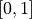之间；

• 该向量的所有元素之和为 1。

### 模型的训练： tf.keras.losses 和 tf.keras.optimizer¶

num_epochs = 5
batch_size = 50
learning_rate = 0.001


model = MLP()


• 将这批数据送入模型，计算出模型的预测值；

• 将模型预测值与真实值进行比较，计算损失函数（loss）。这里使用 tf.keras.losses 中的交叉熵函数作为损失函数；

• 计算损失函数关于模型变量的导数；

• 将求出的导数值传入优化器，使用优化器的 apply_gradients 方法更新模型参数以最小化损失函数（优化器的详细使用方法见 前章 ）。

    num_batches = int(data_loader.num_train_data // batch_size * num_epochs)
for batch_index in range(num_batches):
y_pred = model(X)
loss = tf.keras.losses.sparse_categorical_crossentropy(y_true=y, y_pred=y_pred)
loss = tf.reduce_mean(loss)
print("batch %d: loss %f" % (batch_index, loss.numpy()))


tf.keras 中，有两个交叉熵相关的损失函数 tf.keras.losses.categorical_crossentropytf.keras.losses.sparse_categorical_crossentropy 。其中 sparse 的含义是，真实的标签值 y_true 可以直接传入 int 类型的标签类别。具体而言：

loss = tf.keras.losses.sparse_categorical_crossentropy(y_true=y, y_pred=y_pred)


loss = tf.keras.losses.categorical_crossentropy(
y_true=tf.one_hot(y, depth=tf.shape(y_pred)[-1]),
y_pred=y_pred
)


### 模型的评估： tf.keras.metrics¶

    sparse_categorical_accuracy = tf.keras.metrics.SparseCategoricalAccuracy()
for batch_index in range(num_batches):
start_index, end_index = batch_index * batch_size, (batch_index + 1) * batch_size
print("test accuracy: %f" % sparse_categorical_accuracy.result())


test accuracy: 0.947900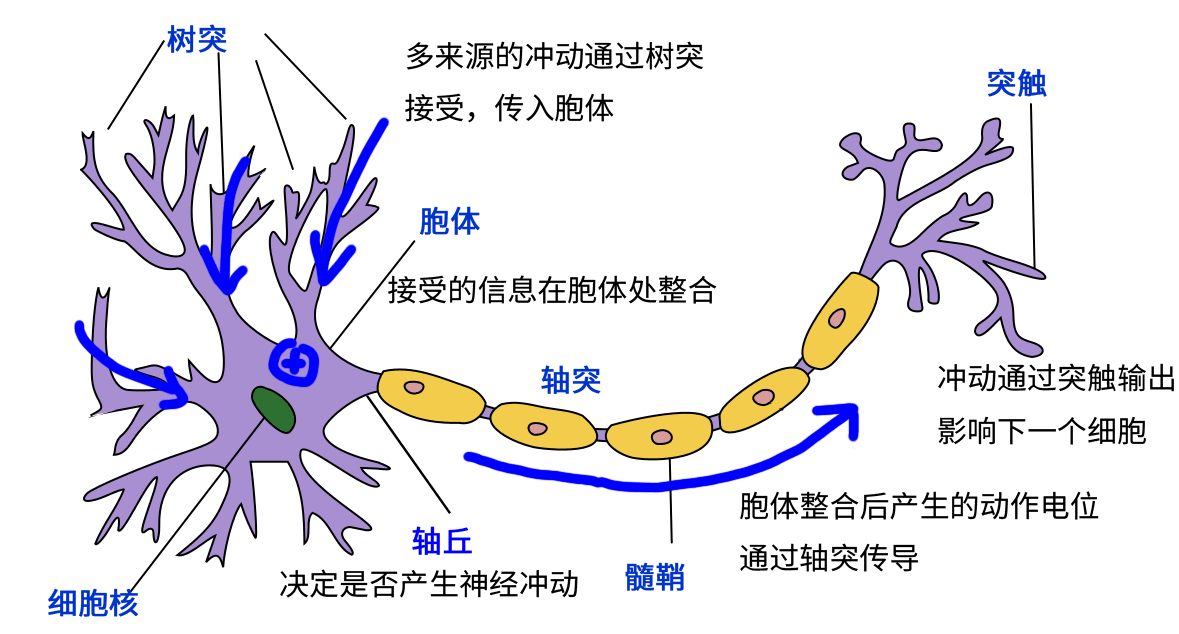3

## 卷积神经网络（CNN）¶

### 使用Keras实现卷积神经网络¶

class CNN(tf.keras.Model):
def __init__(self):
super().__init__()
self.conv1 = tf.keras.layers.Conv2D(
filters=32,             # 卷积层神经元（卷积核）数目
kernel_size=[5, 5],     # 感受野大小
activation=tf.nn.relu   # 激活函数
)
self.pool1 = tf.keras.layers.MaxPool2D(pool_size=[2, 2], strides=2)
self.conv2 = tf.keras.layers.Conv2D(
filters=64,
kernel_size=[5, 5],
activation=tf.nn.relu
)
self.pool2 = tf.keras.layers.MaxPool2D(pool_size=[2, 2], strides=2)
self.flatten = tf.keras.layers.Reshape(target_shape=(7 * 7 * 64,))
self.dense1 = tf.keras.layers.Dense(units=1024, activation=tf.nn.relu)
self.dense2 = tf.keras.layers.Dense(units=10)

def call(self, inputs):
x = self.conv1(inputs)                  # [batch_size, 28, 28, 32]
x = self.pool1(x)                       # [batch_size, 14, 14, 32]
x = self.conv2(x)                       # [batch_size, 14, 14, 64]
x = self.pool2(x)                       # [batch_size, 7, 7, 64]
x = self.flatten(x)                     # [batch_size, 7 * 7 * 64]
x = self.dense1(x)                      # [batch_size, 1024]
x = self.dense2(x)                      # [batch_size, 10]
output = tf.nn.softmax(x)
return output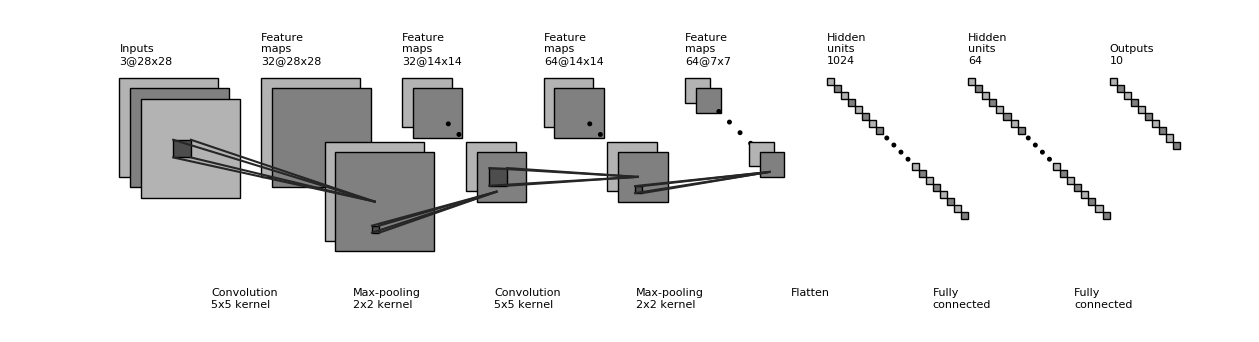test accuracy: 0.988100


### 使用Keras中预定义的经典卷积神经网络结构¶

tf.keras.applications 中有一些预定义好的经典卷积神经网络结构，如 VGG16VGG19ResNetMobileNet 等。我们可以直接调用这些经典的卷积神经网络结构（甚至载入预训练的参数），而无需手动定义网络结构。

model = tf.keras.applications.MobileNetV2()


• input_shape ：输入张量的形状（不含第一维的Batch），大多默认为 224 × 224 × 3 。一般而言，模型对输入张量的大小有下限，长和宽至少为 32 × 3275 × 75

• include_top ：在网络的最后是否包含全连接层，默认为 True

• weights ：预训练权值，默认为 'imagenet' ，即为当前模型载入在ImageNet数据集上预训练的权值。如需随机初始化变量可设为 None

• classes ：分类数，默认为1000。修改该参数需要 include_top 参数为 Trueweights 参数为 None

import tensorflow as tf
import tensorflow_datasets as tfds

num_batches = 1000
batch_size = 50
learning_rate = 0.001

dataset = dataset.map(lambda img, label: (tf.image.resize(img, [224, 224]) / 255.0, label)).shuffle(1024).batch(32)
model = tf.keras.applications.MobileNetV2(weights=None, classes=5)
for images, labels in dataset:
labels_pred = model(images)
loss = tf.keras.losses.sparse_categorical_crossentropy(y_true=labels, y_pred=labels_pred)
loss = tf.reduce_mean(loss)
print("loss %f" % loss.numpy())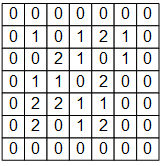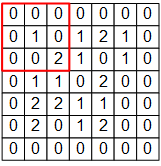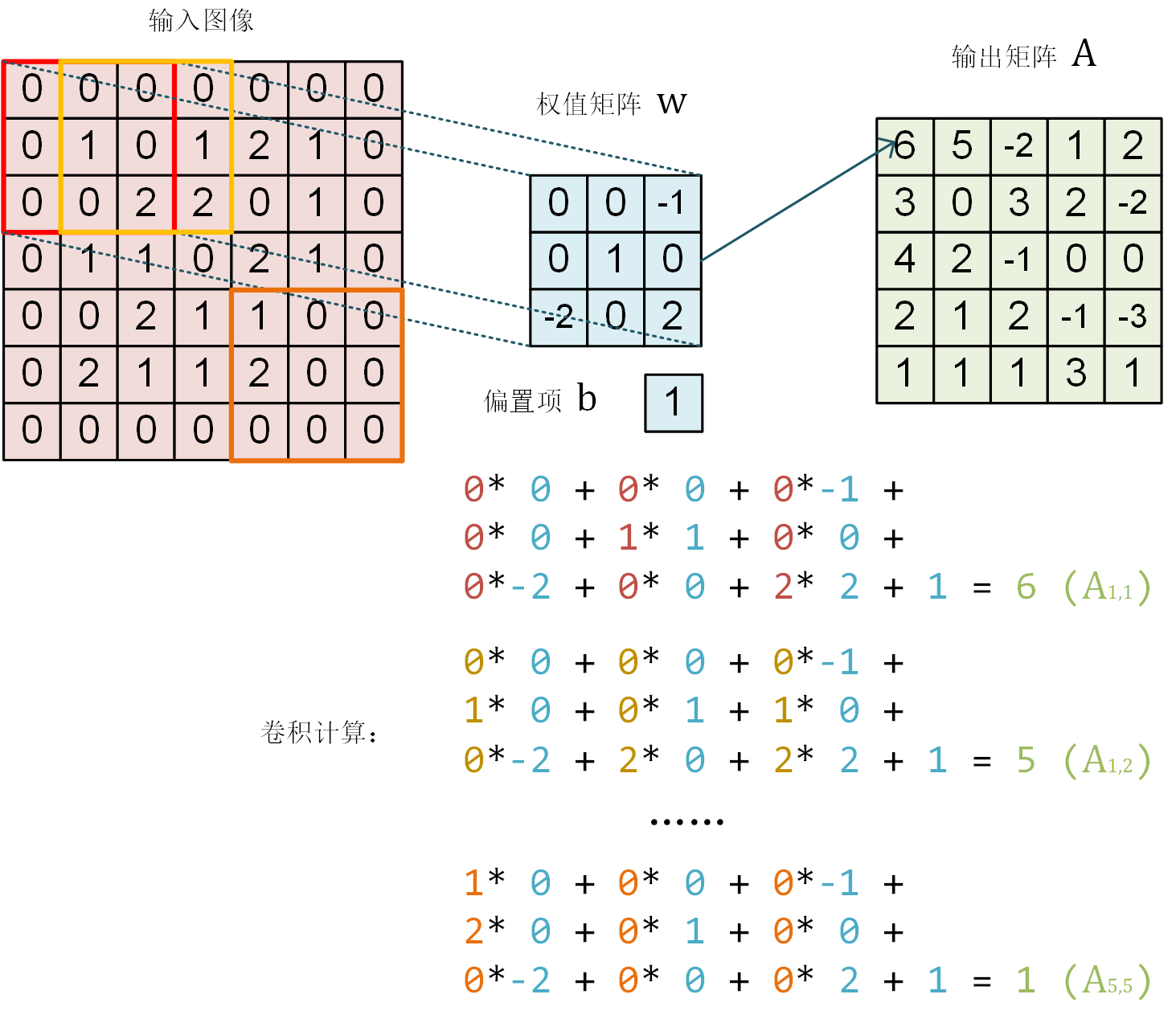# TensorFlow 的图像表示为 [图像数目，长，宽，色彩通道数] 的四维张量
# 这里我们的输入图像 image 的张量形状为 [1, 7, 7, 1]
image = np.array([[
[0, 0, 0, 0, 0, 0, 0],
[0, 1, 0, 1, 2, 1, 0],
[0, 0, 2, 2, 0, 1, 0],
[0, 1, 1, 0, 2, 1, 0],
[0, 0, 2, 1, 1, 0, 0],
[0, 2, 1, 1, 2, 0, 0],
[0, 0, 0, 0, 0, 0, 0]
]], dtype=np.float32)
image = np.expand_dims(image, axis=-1)
W = np.array([[
[ 0, 0, -1],
[ 0, 1, 0 ],
[-2, 0, 2 ]
]], dtype=np.float32)
b = np.array(, dtype=np.float32)


model = tf.keras.models.Sequential([
tf.keras.layers.Conv2D(
filters=1,              # 卷积层神经元（卷积核）数目
kernel_size=[3, 3],     # 感受野大小
kernel_initializer=tf.constant_initializer(W),
bias_initializer=tf.constant_initializer(b)
)]
)


output = model(image)
print(tf.squeeze(output))


tf.Tensor(
[[ 6.  5. -2.  1.  2.]
[ 3.  0.  3.  2. -2.]
[ 4.  2. -1.  0.  0.]
[ 2.  1.  2. -1. -3.]
[ 1.  1.  1.  3.  1.]], shape=(5, 5), dtype=float32)


4

## 循环神经网络（RNN）¶

I am a studen


class DataLoader():
def __init__(self):
path = tf.keras.utils.get_file('nietzsche.txt',
origin='https://s3.amazonaws.com/text-datasets/nietzsche.txt')
with open(path, encoding='utf-8') as f:
self.chars = sorted(list(set(self.raw_text)))
self.char_indices = dict((c, i) for i, c in enumerate(self.chars))
self.indices_char = dict((i, c) for i, c in enumerate(self.chars))
self.text = [self.char_indices[c] for c in self.raw_text]

def get_batch(self, seq_length, batch_size):
seq = []
next_char = []
for i in range(batch_size):
index = np.random.randint(0, len(self.text) - seq_length)
seq.append(self.text[index:index+seq_length])
next_char.append(self.text[index+seq_length])
return np.array(seq), np.array(next_char)       # [batch_size, seq_length], [num_batch]output, state = self.cell(inputs[:, t, :], state) 图示

class RNN(tf.keras.Model):
def __init__(self, num_chars, batch_size, seq_length):
super().__init__()
self.num_chars = num_chars
self.seq_length = seq_length
self.batch_size = batch_size
self.cell = tf.keras.layers.LSTMCell(units=256)
self.dense = tf.keras.layers.Dense(units=self.num_chars)

def call(self, inputs, from_logits=False):
inputs = tf.one_hot(inputs, depth=self.num_chars)       # [batch_size, seq_length, num_chars]
state = self.cell.get_initial_state(batch_size=self.batch_size, dtype=tf.float32)
for t in range(self.seq_length):
output, state = self.cell(inputs[:, t, :], state)
logits = self.dense(output)
if from_logits:
return logits
else:
return tf.nn.softmax(logits)


    num_batches = 1000
seq_length = 40
batch_size = 50
learning_rate = 1e-3


• DataLoader 中随机取一批训练数据；

• 将这批数据送入模型，计算出模型的预测值；

• 将模型预测值与真实值进行比较，计算损失函数（loss）；

• 计算损失函数关于模型变量的导数；

• 使用优化器更新模型参数以最小化损失函数。

    data_loader = DataLoader()
for batch_index in range(num_batches):
y_pred = model(X)
loss = tf.keras.losses.sparse_categorical_crossentropy(y_true=y, y_pred=y_pred)
loss = tf.reduce_mean(loss)
print("batch %d: loss %f" % (batch_index, loss.numpy()))


    def predict(self, inputs, temperature=1.):
batch_size, _ = tf.shape(inputs)
logits = self(inputs, from_logits=True)
prob = tf.nn.softmax(logits / temperature).numpy()
return np.array([np.random.choice(self.num_chars, p=prob[i, :])
for i in range(batch_size.numpy())])


    X_, _ = data_loader.get_batch(seq_length, 1)
for diversity in [0.2, 0.5, 1.0, 1.2]:
X = X_
print("diversity %f:" % diversity)
for t in range(400):
y_pred = model.predict(X, diversity)
X = np.concatenate([X[:, 1:], np.expand_dims(y_pred, axis=1)], axis=-1)
print("\n")


diversity 0.200000:
conserted and conseive to the conterned to it is a self--and seast and the selfes as a seast the expecience and and and the self--and the sered is a the enderself and the sersed and as a the concertion of the series of the self in the self--and the serse and and the seried enes and seast and the sense and the eadure to the self and the present and as a to the self--and the seligious and the enders

diversity 0.500000:
can is reast to as a seligut and the complesed
has fool which the self as it is a the beasing and us immery and seese for entoured underself of the seless and the sired a mears and everyther to out every sone thes and reapres and seralise as a streed liees of the serse to pease the cersess of the selung the elie one of the were as we and man one were perser has persines and conceity of all self-el

diversity 1.000000:
entoles by
their lisevers de weltaale, arh pesylmered, and so jejurted count have foursies as is
descinty iamo; to semplization refold, we dancey or theicks-welf--atolitious on his
such which
here
oth idey of pire master, ie gerw their endwit in ids, is an trees constenved mase commars is leed mad decemshime to the mor the elige. the fedies (byun their ope wopperfitious--antile and the it as the f

diversity 1.200000:
inselfy!--ie the rads incults of to prusely le]enfes patuateded:.--a coud--theiritibaior "nrallysengleswout peessparify oonsgoscess teemind thenry ansken suprerial mus, cigitioum: 4reas. whouph: who
eved
arn inneves to sya" natorne. hag open reals whicame oderedte,[fingo is
zisternethta simalfule dereeg hesls lang-lyes thas quiin turjentimy; periaspedey tomm--whach

5

• 对输入向量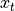通过矩阵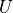进行线性变换，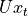与状态 s 具有相同的维度；

•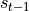通过矩阵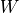进行线性变换，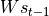与状态 s 具有相同的维度；

• 将上述得到的两个向量相加并通过激活函数，作为当前状态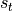的值，即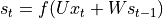。也就是说，当前状态的值是上一个状态的值和当前输入进行某种信息整合而产生的；

• 对当前状态通过矩阵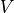进行线性变换，得到当前时刻的输出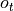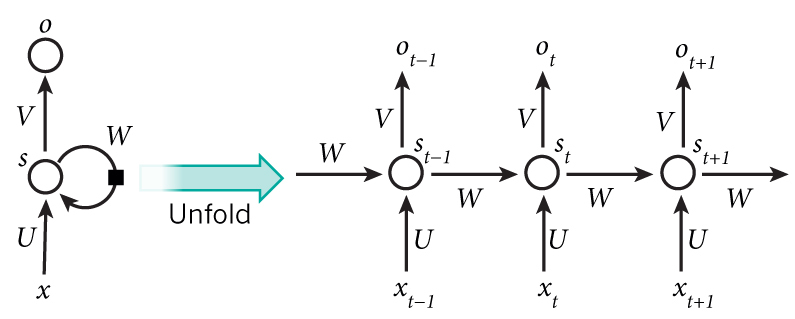## 深度强化学习（DRL）¶

import gym

env = gym.make('CartPole-v1')       # 实例化一个游戏环境，参数为游戏名称
state = env.reset()                 # 初始化环境，获得初始状态
while True:
env.render()                    # 对当前帧进行渲染，绘图到屏幕
action = model.predict(state)   # 假设我们有一个训练好的模型，能够通过当前状态预测出这时应该进行的动作
next_state, reward, done, info = env.step(action)   # 让环境执行动作，获得执行完动作的下一个状态，动作的奖励，游戏是否已结束以及额外信息
if done:                        # 如果游戏结束则退出循环
break


import tensorflow as tf
import numpy as np
import gym
import random
from collections import deque

num_episodes = 500              # 游戏训练的总episode数量
num_exploration_episodes = 100  # 探索过程所占的episode数量
max_len_episode = 1000          # 每个episode的最大回合数
batch_size = 32                 # 批次大小
learning_rate = 1e-3            # 学习率
gamma = 1.                      # 折扣因子
initial_epsilon = 1.            # 探索起始时的探索率
final_epsilon = 0.01            # 探索终止时的探索率


class QNetwork(tf.keras.Model):
def __init__(self):
super().__init__()
self.dense1 = tf.keras.layers.Dense(units=24, activation=tf.nn.relu)
self.dense2 = tf.keras.layers.Dense(units=24, activation=tf.nn.relu)
self.dense3 = tf.keras.layers.Dense(units=2)

def call(self, inputs):
x = self.dense1(inputs)
x = self.dense2(x)
x = self.dense3(x)
return x

def predict(self, inputs):
q_values = self(inputs)
return tf.argmax(q_values, axis=-1)


if __name__ == '__main__':
env = gym.make('CartPole-v1')       # 实例化一个游戏环境，参数为游戏名称
model = QNetwork()
replay_buffer = deque(maxlen=10000) # 使用一个 deque 作为 Q Learning 的经验回放池
epsilon = initial_epsilon
for episode_id in range(num_episodes):
state = env.reset()             # 初始化环境，获得初始状态
epsilon = max(                  # 计算当前探索率
initial_epsilon * (num_exploration_episodes - episode_id) / num_exploration_episodes,
final_epsilon)
for t in range(max_len_episode):
env.render()                                # 对当前帧进行渲染，绘图到屏幕
if random.random() < epsilon:               # epsilon-greedy 探索策略，以 epsilon 的概率选择随机动作
action = env.action_space.sample()      # 选择随机动作（探索）
else:
action = model.predict(np.expand_dims(state, axis=0)).numpy()   # 选择模型计算出的 Q Value 最大的动作
action = action

# 让环境执行动作，获得执行完动作的下一个状态，动作的奖励，游戏是否已结束以及额外信息
next_state, reward, done, info = env.step(action)
# 如果游戏Game Over，给予大的负奖励
reward = -10. if done else reward
# 将(state, action, reward, next_state)的四元组（外加 done 标签表示是否结束）放入经验回放池
replay_buffer.append((state, action, reward, next_state, 1 if done else 0))
# 更新当前 state
state = next_state

if done:                                    # 游戏结束则退出本轮循环，进行下一个 episode
print("episode %d, epsilon %f, score %d" % (episode_id, epsilon, t))
break

if len(replay_buffer) >= batch_size:
# 从经验回放池中随机取一个批次的四元组，并分别转换为 NumPy 数组
batch_state, batch_action, batch_reward, batch_next_state, batch_done = zip(
*random.sample(replay_buffer, batch_size))
batch_state, batch_reward, batch_next_state, batch_done = \
[np.array(a, dtype=np.float32) for a in [batch_state, batch_reward, batch_next_state, batch_done]]
batch_action = np.array(batch_action, dtype=np.int32)

q_value = model(batch_next_state)
y = batch_reward + (gamma * tf.reduce_max(q_value, axis=1)) * (1 - batch_done)  # 计算 y 值
loss = tf.keras.losses.mean_squared_error(  # 最小化 y 和 Q-value 的距离
y_true=y,
y_pred=tf.reduce_sum(model(batch_state) * tf.one_hot(batch_action, depth=2), axis=1)
)


• 砖块的颜色信息并不是很重要，画面转换成灰度也不影响操作，因此可以去除状态中的颜色信息（即将图片转为灰度表示）；

• 小球移动的信息很重要，如果只知道单帧画面而不知道小球往哪边运动，即使是人也很难判断挡板应当移动的方向。因此，必须在状态中加入表征小球运动方向的信息。一个简单的方式是将当前帧与前面几帧的画面进行叠加，得到一个 210 * 160 * X （X 为叠加帧数）的状态表示；

• 每帧的分辨率不需要特别高，只要能大致表征方块、小球和挡板的位置以做出决策即可，因此对于每帧的长宽可做适当压缩。

TODO

## Keras Pipeline *¶

### Keras Sequential/Functional API 模式建立模型¶

        model = tf.keras.models.Sequential([
tf.keras.layers.Flatten(),
tf.keras.layers.Dense(100, activation=tf.nn.relu),
tf.keras.layers.Dense(10),
tf.keras.layers.Softmax()
])


        inputs = tf.keras.Input(shape=(28, 28, 1))
x = tf.keras.layers.Flatten()(inputs)
x = tf.keras.layers.Dense(units=100, activation=tf.nn.relu)(x)
x = tf.keras.layers.Dense(units=10)(x)
outputs = tf.keras.layers.Softmax()(x)
model = tf.keras.Model(inputs=inputs, outputs=outputs)


### 使用 Keras Model 的 compile 、 fit 和 evaluate 方法训练和评估模型¶

    model.compile(
loss=tf.keras.losses.sparse_categorical_crossentropy,
metrics=[tf.keras.metrics.sparse_categorical_accuracy]
)


tf.keras.Model.compile 接受 3 个重要的参数：

• oplimizer ：优化器，可从 tf.keras.optimizers 中选择；

• loss ：损失函数，可从 tf.keras.losses 中选择；

• metrics ：评估指标，可从 tf.keras.metrics 中选择。

    model.fit(data_loader.train_data, data_loader.train_label, epochs=num_epochs, batch_size=batch_size)


tf.keras.Model.fit 接受 5 个重要的参数：

• x ：训练数据；

• y ：目标数据（数据标签）；

• epochs ：将训练数据迭代多少遍；

• batch_size ：批次的大小；

• validation_data ：验证数据，可用于在训练过程中监控模型的性能。

Keras 支持使用 tf.data.Dataset 进行训练，详见 tf.data

    print(model.evaluate(data_loader.test_data, data_loader.test_label))


## 自定义层、损失函数和评估指标 *¶

### 自定义层¶

class MyLayer(tf.keras.layers.Layer):
def __init__(self):
super().__init__()
# 初始化代码

def build(self, input_shape):     # input_shape 是一个 TensorShape 类型对象，提供输入的形状
# 在第一次使用该层的时候调用该部分代码，在这里创建变量可以使得变量的形状自适应输入的形状
# 而不需要使用者额外指定变量形状。
# 如果已经可以完全确定变量的形状，也可以在__init__部分创建变量

def call(self, inputs):
# 模型调用的代码（处理输入并返回输出）
return output


class LinearLayer(tf.keras.layers.Layer):
def __init__(self, units):
super().__init__()
self.units = units

def build(self, input_shape):     # 这里 input_shape 是第一次运行call()时参数inputs的形状
shape=[input_shape[-1], self.units], initializer=tf.zeros_initializer())
shape=[self.units], initializer=tf.zeros_initializer())

def call(self, inputs):
y_pred = tf.matmul(inputs, self.w) + self.b
return y_pred


class LinearModel(tf.keras.Model):
def __init__(self):
super().__init__()
self.layer = LinearLayer(units=1)

def call(self, inputs):
output = self.layer(inputs)
return output


### 自定义损失函数和评估指标¶

class MeanSquaredError(tf.keras.losses.Loss):
def call(self, y_true, y_pred):
return tf.reduce_mean(tf.square(y_pred - y_true))


class SparseCategoricalAccuracy(tf.keras.metrics.Metric):
def __init__(self):
super().__init__()

def update_state(self, y_true, y_pred, sample_weight=None):
values = tf.cast(tf.equal(y_true, tf.argmax(y_pred, axis=-1, output_type=tf.int32)), tf.int32)

def result(self):
return self.count / self.total

LeCun1998
1. LeCun, L. Bottou, Y. Bengio, and P. Haffner. “Gradient-based learning applied to document recognition.” Proceedings of the IEEE, 86(11):2278-2324, November 1998. http://yann.lecun.com/exdb/mnist/

Graves2013

Graves, Alex. “Generating Sequences With Recurrent Neural Networks.” ArXiv:1308.0850 [Cs], August 4, 2013. http://arxiv.org/abs/1308.0850.

Mnih2013

Mnih, Volodymyr, Koray Kavukcuoglu, David Silver, Alex Graves, Ioannis Antonoglou, Daan Wierstra, and Martin Riedmiller. “Playing Atari with Deep Reinforcement Learning.” ArXiv:1312.5602 [Cs], December 19, 2013. http://arxiv.org/abs/1312.5602.